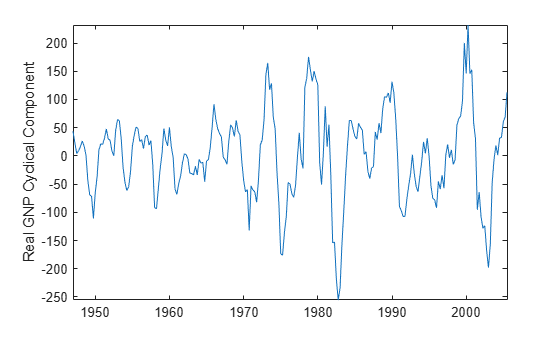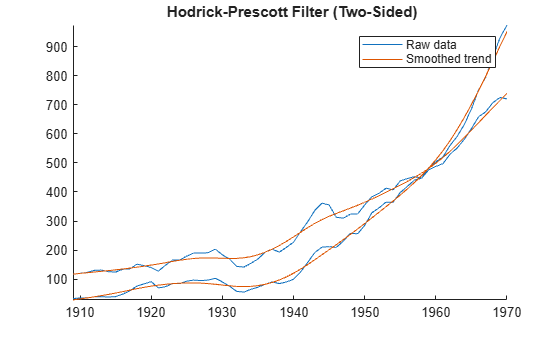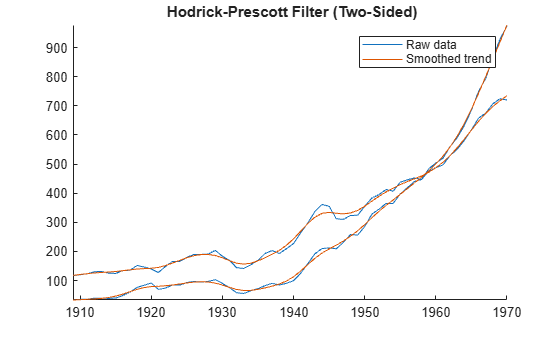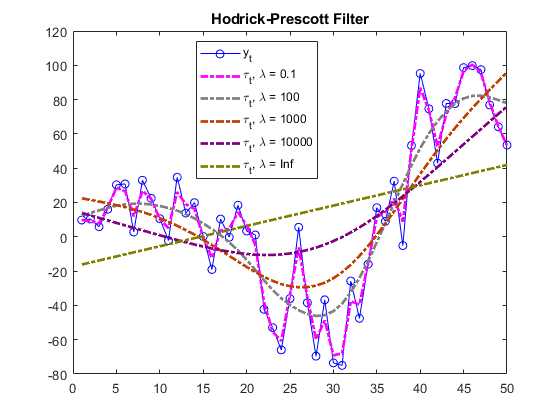# hpfilter

Hodrick-Prescott filter for trend and cyclical components

## Syntax

[Trend,Cyclical] = hpfilter(Y)
[TTbl,CTbl] = hpfilter(Tbl)
[___] = hpfilter(___,Name=Value)
hpfilter(___)
hpfilter(ax,___)
[___,h] = hpfilter(___)

## Description

Separate one or more time series into trend and cyclical components by applying the Hodrick-Prescott filter . hpfilter optionally plots the series and trend component, with cycles removed. The plot helps you select a smoothing parameter.

example

[Trend,Cyclical] = hpfilter(Y) returns the trend Trend and cyclical Cycilcal components from applying the Hodrick-Prescott Filter to each variable (column) of the input matrix of time series data Y. The smoothing parameter default is 1600, suggested in  for quarterly data.

example

[TTbl,CTbl] = hpfilter(Tbl) returns the tables or timetables TTbl and CTbl containing variables for the trend and cyclical components, respectively, from applying the Hodrick-Prescott Filter to each variable in the input table or timetable Tbl. To select different variables in Tbl to filter, use the DataVariables name-value argument.

example

[___] = hpfilter(___,Name=Value) specifies options using one or more name-value arguments in addition to any of the input argument combinations in previous syntaxes. hpfilter returns the output argument combination for the corresponding input arguments. For example, hpfilter(Tbl,Smoothing=100,DataVariables=1:5) applies the Hodrick-Prescott filter to the first five variables in the input table Tbl and sets the smoothing parameter to 100.

example

hpfilter(___) plots time series variables in the input data and their respective trend components, computed by the Hodrick-Prescott filter, on the same axes.
hpfilter(ax,___) plots on the axes specified by ax instead of the current axes (gca). ax can precede any of the input argument combinations in the previous syntaxes.

example

[___,h] = hpfilter(___) plots the specified series and their trend components, and additionally returns handles to plotted graphics objects. Use elements of h to modify properties of the plot after you create it.

## Examples

collapse all

Plot the cyclical component of the US post-WWII, seasonally adjusted, quarterly, real gross national product (GNPR).

load Data_GNP GNPR = DataTable.GNPR; [trend,cyclical] = hpfilter(GNPR); T = numel(trend)
T = 235 

trend and cyclical are 235-by-1 vectors containing the trend and cyclical components, respectively, resulting from applying the Hodrick-Prescott filter to the series with default smoothing parameter 1600.

plot(dates,cyclical) axis tight ylabel("Real GNP Cyclical Component")Apply the Hodrick-Prescott filter to all variables in input tabular variables.

Load the US equity indices data set, which contains the table DataTable of daily closing prices of the NYSE and NASDAQ composite indices from 1990 through 2011.

load Data_EquityIdx

Create a timetable from the table.

dates = datetime(dates,ConvertFrom="datenum"); TT = table2timetable(DataTable,RowTimes=dates); TT.Dates = [];

Aggregate the daily data in the timetable to quarterly.

TTQ = convert2quarterly(TT);

TTQ contains the closing prices in TT at the end of each quarter.

Apply the Hodrick-Prescott filter to all variables in the quarterly timetable. The default smoothing parameter value is 1600. Display the last few observed components.

[TQTbl,CQTbl] = hpfilter(TTQ); size(TQTbl)
ans = 1×2 48 2 
tail(TQTbl)
 Time NYSE NASDAQ ___________ ______ ______ 31-Mar-2000 605.09 2610.6 30-Jun-2000 614.21 2653.5 30-Sep-2000 622.64 2686.6 31-Dec-2000 630.51 2711.6 31-Mar-2001 637.95 2730.9 30-Jun-2001 645.11 2746.7 30-Sep-2001 652.11 2760.7 31-Dec-2001 659.07 2774.2 
tail(CQTbl)
 Time NYSE NASDAQ ___________ _______ _______ 31-Mar-2000 42.608 1962.3 30-Jun-2000 28.724 1312.6 30-Sep-2000 40.398 986.2 31-Dec-2000 26.359 -241.11 31-Mar-2001 -42.288 -890.66 30-Jun-2001 -23.346 -585.48 30-Sep-2001 -108.27 -1261.9 31-Dec-2001 -69.269 -823.78 

TQTbl and CQTbl are 48-by-2 timetables containing the trend and cyclical components, respectively, of the series in TTQ. Variables in the input and output timetables correspond.

By default, hpfilter filters all variables in the input table or timetable. To select a subset of variables, set the DataVariables option.

To compare outputs, apply the Hodrick-Prescott filter to all variables in the table DataTable and the timetable TT.

% Table input of daily data [DTTTbl,DTCTbl] = hpfilter(DataTable); size(DTTTbl)
ans = 1×2 3028 2 
tail(DTTTbl)
 NYSE NASDAQ ______ ______ 19-Dec-2001 583.83 1977.3 20-Dec-2001 584.4 1976.4 21-Dec-2001 585.02 1975.3 24-Dec-2001 585.67 1974.2 26-Dec-2001 586.35 1973.1 27-Dec-2001 587.04 1972 28-Dec-2001 587.74 1970.8 31-Dec-2001 588.44 1969.7 
tail(DTCTbl)
 NYSE NASDAQ _______ _______ 19-Dec-2001 1.2948 5.5523 20-Dec-2001 -1.1413 -57.834 21-Dec-2001 0.67903 -29.492 24-Dec-2001 0.37592 -29.745 26-Dec-2001 2.2792 -12.408 27-Dec-2001 5.0385 4.4419 28-Dec-2001 6.6419 16.425 31-Dec-2001 1.3645 -19.279 
% Timetable input of daily data [TTbl,CTbl] = hpfilter(TT); size(TTbl)
ans = 1×2 3028 2 
tail(TTbl)
 Time NYSE NASDAQ ___________ ______ ______ 19-Dec-2001 583.83 1977.3 20-Dec-2001 584.4 1976.4 21-Dec-2001 585.02 1975.3 24-Dec-2001 585.67 1974.2 26-Dec-2001 586.35 1973.1 27-Dec-2001 587.04 1972 28-Dec-2001 587.74 1970.8 31-Dec-2001 588.44 1969.7 
tail(CTbl)
 Time NYSE NASDAQ ___________ _______ _______ 19-Dec-2001 1.2948 5.5523 20-Dec-2001 -1.1413 -57.834 21-Dec-2001 0.67903 -29.492 24-Dec-2001 0.37592 -29.745 26-Dec-2001 2.2792 -12.408 27-Dec-2001 5.0385 4.4419 28-Dec-2001 6.6419 16.425 31-Dec-2001 1.3645 -19.279 

Because the data is unaggregated, the outputs of the daily data have more rows than from the quarterly data. The filter results of the daily inputs are equal among the corresponding outputs, but hpfilter returns tables of results, instead of timetables, when you supply data in a table.

Load the Nelson-Plosser macroeconomic data set Data_NelsonPlosser.mat, which contains series measured yearly in the table DataTable.

load Data_NelsonPlosser

Filter the real and nominal GNP series, GNPR and GNPN, respectively. Plot the trend component with each series by additionally returning the vector of graphics objects. Set the smoothing parameter to 2000.

[TTbl,CTbl,h] = hpfilter(DataTimeTable,Smoothing=2000, ... DataVariables=["GNPR" "GNPN"]);Experiment with the smoothing parameter value by filtering the series several more times and setting the smoothing parameter to 0, 10, 100, 1000, 10,000, and Inf. Plot each set of results by not returning any outputs.

smoothing = [10.^(0:4) Inf]; tiledlayout(2,3) for j = 1:numel(smoothing) nexttile hpfilter(DataTimeTable,Smoothing=smoothing(j), ... DataVariables=["GNPR" "GNPN"]); title("\lambda = " + string(smoothing(j))); legend("off") end## Input Arguments

collapse all

Time series data, specified as a numObs-by-numVars numeric matrix. Each column of Y corresponds to a variable, and each row corresponds to an observation.

Data Types: double

Time series data, specified as a table or timetable with numObs rows. Each row of Tbl is an observation.

Specify numVars variables to filter by using the DataVariables argument. The selected variables must be numeric.

Axes on which to plot, specified as an Axes object.

By default, hpfilter plots to the current axes (gca).

Note

hpfilter removes, from the specified data, all rows containing at least one missing observation, represented by a NaN value.

### Name-Value Arguments

Specify optional pairs of arguments as Name1=Value1,...,NameN=ValueN, where Name is the argument name and Value is the corresponding value. Name-value arguments must appear after other arguments, but the order of the pairs does not matter.

Before R2021a, use commas to separate each name and value, and enclose Name in quotes.

Example: hpfilter(Tbl,Smoothing=100,DataVariables=1:5) applies the Hodrick-Prescott filter to the first five variables in the input table Tbl and sets the smoothing parameter to 100.

Trend component smoothing parameter, specified as a nonnegative numeric scalar or vector of length numVars.

For a scalar, hpfilter applies Smoothing to all specified input series. For a vector, hpfilter applies Smoothing(k) to specified input series k in the data.

If Smoothing(k) = 0, hpfilter does not smooth the corresponding trend components. In this case, these conditions apply:

• Trend(:,k) = yk, where yk is the input data of specified variable k.

• Cyclical(:,k) = zeros(numObs,1).

If Smoothing(k) = Inf, hpfilter applies maximum smoothing. In this case, these conditions apply:

• Trend(:,k) is the linear time trend computed by least squares.

• Cyclical(:,k) is the detrended series.

As the magnitude of the smoothing parameter increases, Trend approaches the linear time trend.

Tip

1] suggests values for the smoothing parameter that depend upon

the periodicity of the data:

The smoothing parameter value depends on the periodicity of the data. Although a best practice is to experiment with smoothing values for your data, these values are recommended in :

• 14400 for monthly data

• 1600 for quarterly data

• 100 for yearly data

Example: Smoothing=100

Data Types: double

Variables in Tbl that hpfilter filters, specified as a string vector or cell vector of character vectors containing variable names in Tbl.Properties.VariableNames, or an integer or logical vector representing the indices of names. The selected variables must be numeric.

Example: DataVariables=["GDP" "CPI"]

Example: DataVariables=[true true false false] or DataVariables=[1 2] selects the first and second table variables.

Data Types: double | logical | char | cell | string

## Output Arguments

collapse all

Trend component τt of each series in the data, returned as a numObs-by-numVars numeric matrix. hpfilter returns Trend when you supply the input Y.

Cyclical component ct of each series in the data, returned as a numObs-by-numVars numeric matrix. hpfilter returns Cyclical when you supply the input Y.

Trend component τt of each specified series, returned as a numObs-by-numVars table or timetable, the same data type as Tbl. hpfilter returns TTbl when you supply the input Tbl.

Cyclical component ct of each specified series, returned as a numObs-by-numVars table or timetable, the same data type as Tbl. hpfilter returns CTbl when you supply the input Tbl.

Handles to plotted graphics objects, returned as a vector of graphics objects. hpfilter plots the data and trend only when you return no outputs or you return h.

h contains unique plot identifiers, which you can use to query or modify properties of the plot.

collapse all

### Hodrick-Prescott Filter

The Hodrick-Prescott filter decomposes an observed time series yt (Y) into a trend component τt (Trend) and a cyclical component ct (Cyclical) such that yt = τt + ct.

The objective function of the filter is

$f\left({\tau }_{t}\right)=\sum _{t=1}^{T}{\left({y}_{t}-{\tau }_{t}\right)}^{2}+\lambda \sum _{t=2}^{T-1}{\left[\left({\tau }_{t+1}-{\tau }_{t}\right)-\left({\tau }_{t}-{\tau }_{t-1}\right)\right]}^{2},$

where:

The programming problem is to minimize the objective function over τ1,…,τT. The objective penalizes the sum of squares for the cyclical component with the sum of squares of second-order differences for the trend component (trend acceleration penalty). If λ = 0, the minimum of the objective is 0 with τt = yt for all t. As λ increases, the penalty for a flexible trend increases, resulting in an increasingly smoother trend. When λ is arbitrarily large, the trend acceleration approaches 0, resulting in a linear trend.

This figure shows the effects of increasing the smoothing parameter on the trend component for a simulated series.The filter is equivalent to a cubic spline smoother, where the smoothed component is τt.

## Tips

• For high-frequency series, the Hodrick-Prescott filter can produce anomalous endpoint effects. In this case, do not extrapolate the series using the results of the filter.

 Hodrick, Robert J., and Edward C. Prescott. "Postwar U.S. Business Cycles: An Empirical Investigation." Journal of Money, Credit and Banking 29, no. 1 (February 1997): 1–16. https://doi.org/10.2307/2953682.